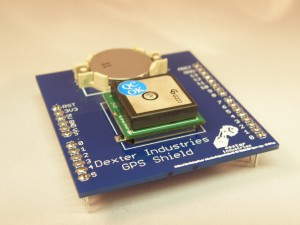# Arduino GPS Project: Converting CoordinatesThis page is about how to convert GPS coordinates from a standard GPS (GPRMC string data) into decimal-degree coordinates in the Arduino language.

An example code is given below for how to take in a a float in the GPS format and convert it into decimal-degrees coordinates (dd.mmssss). This is written in Arduino language and is for GPS coordinates in arduino projects.

You may have an Arduino GPS Project that requires you to use GPS coordinates. GPS coordinates come in the format (ddmm.ssss . . . degree degree minute minute POINT second second second second). These coordinates need to be converted into decimal-degree coordinates (dd.mmssss . . . degree degree POINT minute minute second second second second). Decimal Degree coordinates are used in Google Maps for example.

Below is some example code for converting a number that is in GPS-style to decimal-degree style floating point number.  This example can be used in your Arduino GPS project by copying and pasting.

This code was specifically designed, and is used, in Dexter Industries Arduino GPS Shield.

## Converting Coordinates from GPS-style (ddmm.ssss) to Decimal Style (dd.mmssss) in Arduino

```float conv_coords(float in_coords)
{
//Initialize the location.
float f = in_coords;
// Get the first two digits by turning f into an integer, then doing an integer divide by 100;
// firsttowdigits should be 77 at this point.
int firsttwodigits = ((int)f)/100; //This assumes that f < 10000.
float nexttwodigits = f - (float)(firsttwodigits*100);
float theFinalAnswer = (float)(firsttwodigits + nexttwodigits/60.0);